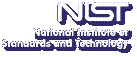Up# Clique Number and Chromatic Number of Graphs Defined by Convex Geometries

Walter Morris
Department of Mathematical Sciences, George Mason University

Thursday, October 18, 2012 15:00-16:00,
Building 101, Lecture Room C
Gaithersburg
Thursday, October 18, 2012 13:00-14:00,
Room 1-4058
Boulder

Abstract:

Given a finite set $X$ of points in general position in the plane, one can define the copoint graph ${\cal G}(X)$, a graph with at most $n^2$ vertices that has the property that its $k$-cliques correspond to sets of $k$ points in $X$ that form vertex sets of convex $n$-gons. An old conjecture of Erdos and Szekeres is that if $X$ does not contain the vertex set of a convex $k$-gon, then $|X| \le 2^{k-2}$. We proved in 2006 that if the chromatic number of ${\cal G}(X)$ is less than $k$, then $|X| \le 2^{k-2}$. In trying to understand the relationship of clique number and chromatic number of copoint graphs, we are led to abstract convex geometries, for which the graph ${\cal G}$ can also be defined, but which provide a larger variety of graphs to study.

This is joint work with Ph.D. student Jonathan Beagley.

Speaker Bio: Walter Morris received his Ph.D. in Operations Research from Cornell University in 1986 under the direction of Mike Todd. He is currently Professor and Coordinator of the Graduate Program in Mathematical Sciences at George Mason University. His research interests are in Computational Geometry and Optimization.

Presentation Slides: PDF

Contact: B. Cloteaux

Note: Visitors from outside NIST must contact Cathy Graham; (301) 975-3668; at least 24 hours in advance.